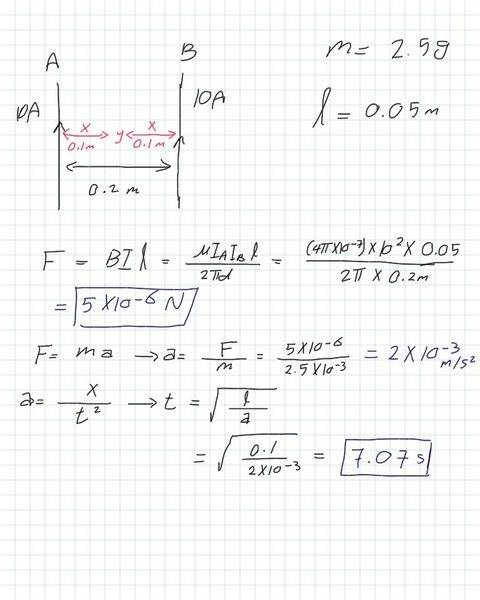# When will two current-carrying wires touch each other

## Homework Statement

Two wires are free to move, the current in each one is 10 A, the mass of each one is 2.5 g, they are separated by a distance of 20 cm, and the length of each one is 5 cm.
Determine (i) where and (ii) when will the two wire touch each other if they were standing parallel to each other.

## Homework Equations

1. B=μi/2πd
2. F= Bil
3. F (interchangeable between two wires) =μi₁i₂l/2πd
4. a= Δv/t = Δx/t²

(Where: B is the magnetic flux density, i is the current, F is the force, a is the acceleration, v is the velocity, x is the distance and t is the time.)

## The Attempt at a Solution

[/B]
(i) where will they touch each other?
Since the direction of the current is not mentioned in the problem statement, I assumed that the current in both wires would be in the same direction so that they can attract each other.
And since the current in both wires is 10A, and it flows in the same direction, they will touch each other at half the distance between the wires (at point y in Figure below).

(ii) when will they touch each other?
That's my attempt to determine the time they reach point y.Note: Δx= 0.1-0=0.1 =x.
(Ia and Ib) are (i₁ and i₂).
So, is my attempt correct?

cnh1995
Homework Helper
Gold Member
Have you studied calculus?
The force is not constant throughout. You need to use differential equations.

Have you studied calculus?
The force is not constant throughout. You need to use differential equations.

cnh1995
Homework Helper
Gold Member
I gather you are a high school student from your profile. I now doubt that you are supposed to use DEs. Have you formally studied calculus? What is the provided answer?

Maybe you should just assume the force to be constant.

I gather you are a high school student from your profile. I now doubt that you are supposed to use DEs. Have you formally studied calculus? What is the provided answer?

Maybe you should just assume the force to be constant.
I studied calculus two years ago. This year I only have physics, chemistry, biology and geology. I remember only a small bit of information about differential equations, because I don't use them in my physics course this year.
There is no a provided answer unfortunately.
And yes, I guess I should assume that the force is constant. So, how would be my attempt at solution putting those points into consideration.

cnh1995
Homework Helper
Gold Member
Have you studied calculus?
The force is not constant throughout. You need to use differential equations.
Scratch that!
(Even I'm not sure if I can solve it using DEs).

So, how would be my attempt at solution putting those points into consideration.
I didn't check the numbers but your method looks good to me.

Scratch that!
(Even I'm not sure if I can solve it using DEs).

I didn't check the numbers but your method looks good to me.
Looks?!! But, cnh1995, I depend on your help to pass my physics exam this year. I have no teacher and that problem confused me, could you check the numbers, please, and give me the final word.
Thanks in advanceLast edited:
cnh1995
Homework Helper
Gold Member
Looks?!! But, cnh1995, I depend on your help to pass my physics exam this year. I have no teacher and this problem confused me, could you check the numbers, please, and give me the final word.
Thanks in advanceI am getting the same answers.

Ok, thank you very much!

cnh1995
Homework Helper
Gold Member
Ok, thank you very much!
You're welcome!